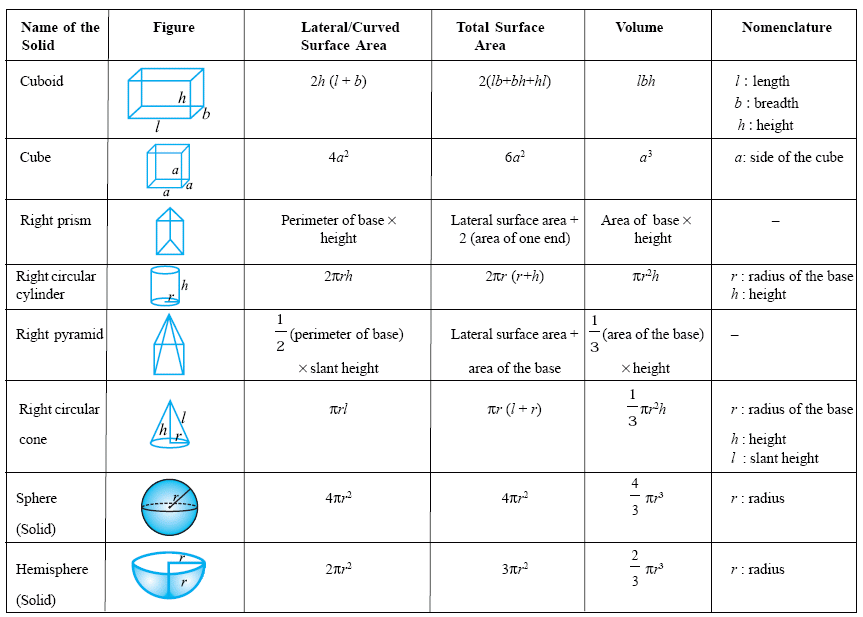kwizNET Subscribers, please login to turn off the Ads!
 Email us to get an instant 20% discount on highly effective K-12 Math & English kwizNET Programs!

#### Online Quiz (WorksheetABCD)

Questions Per Quiz = 2 4 6 8 10

### Geometry6.1 Geometry Formulas Summary#### Areas

square = a2
rectangle = ab
circle = p r2
ellipse = p r1 r2
triangle = (1/2) b h
equilateral triangle = Ö3/4 a2
triangle given SAS = (1/2) a b sin C
triangle given a,b,c = Ö[s(s-a)(s-b)(s-c)]
when s = (a+b+c)/2 (Heron's formula)
parallelogram = bh
trapezoid = h/2 (b1 + b2)
regular polygon = (1/2) n sin(360°/n) a2
when n = # of sides and a = length from center to a corner

#### Volumes

cube = a3
rectangular prism = a b c
irregular prism = b h
cylinder = b h = p r2h
pyramid = (1/3) b h
cone = (1/3) b h = 1/3 [p r2 h ]
sphere = (4/3) p r3
ellipsoid = (4/3) p r1 r2 r3
Directions: Memorize the above formulae and have someone test your knowledge. Write all the formulas on a sheet of paper.

#### Subscription to kwizNET Learning System offers the following benefits:

• Unrestricted access to grade appropriate lessons, quizzes, & printable worksheets
• Instant scoring of online quizzes
• Progress tracking and award certificates to keep your student motivated
• Unlimited practice with auto-generated 'WIZ MATH' quizzes
• Child-friendly website with no advertisements
• Choice of Math, English, Science, & Social Studies Curriculums
• Excellent value for K-12 and ACT, SAT, & TOEFL Test Preparation
• Get discount offers by sending an email to discounts@kwiznet.com

 Quiz Timer